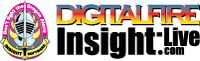•The secret to cool bodies and glazes is a lot of testing.
•The secret to know what to test is material and chemistry knowledge.
•The secret to learning from testing is documentation.
•The place to test, do the chemistry and document is an account at https://insight-live.com
•The place to get the knowledge is https://digitalfire.com

Sign-up at https://insight-live.com today.

# Arcilla de Pontezuelo (Cuba)

## Cuban clay

 Oxide Analysis Formula CaO 0.39% 0.030 MgO 0.63% 0.068 K2O 2.07% 0.096 Na2O 0.37% 0.026 TiO2 0.65% 0.035 Al2O3 23.45% 1.000 SiO2 64.80% 4.690 Fe2O3 0.42% 0.011 LOI 6.44 Oxide Weight 403.56 Formula Weight 431.34

## XML for Import into INSIGHT

<?xml version="1.0" encoding="UTF-8"?> <material name="Arcilla de Pontezuelo (Cuba)" descrip="Cuban clay" searchkey="" loi="0.00" casnumber=""> <oxides> <oxide symbol="CaO" name="Calcium Oxide, Calcia" status="" percent="0.390" tolerance=""/> <oxide symbol="MgO" name="Magnesium Oxide, Magnesia" status="" percent="0.630" tolerance=""/> <oxide symbol="K2O" name="Potassium Oxide" status="" percent="2.070" tolerance=""/> <oxide symbol="Na2O" name="Sodium Oxide, Soda" status="" percent="0.370" tolerance=""/> <oxide symbol="TiO2" name="Titanium Dioxide, Titania" status="" percent="0.650" tolerance=""/> <oxide symbol="Al2O3" name="Aluminum Oxide, Alumina" status="" percent="23.450" tolerance=""/> <oxide symbol="SiO2" name="Silicon Dioxide, Silica" status="" percent="64.800" tolerance=""/> <oxide symbol="Fe2O3" name="Iron Oxide, Ferric Oxide" status="" percent="0.420" tolerance=""/> </oxides> <volatiles> <volatile symbol="LOI" name="Loss on Ignition" percent="6.440" tolerance=""/> </volatiles> </material>